## Lorentz Transformation

A 4-D transformation satisfied by all Four-Vectors,(1)

In the theory of special relativity,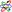the Lorentz transformation replaces the Galilean Transformation as the valid transformation law between reference frames moving with respect to one another at constant Velocity. Letbe the Position Four-Vector with, and let the relative motion be along the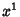axis with Velocity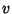. Then (1) becomes(2)

where the Lorentz Tensor is given by(3)

Here,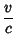(4)(5)

Written explicitly, the transformation betweenandcoordinate is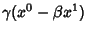(6)(7)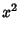(8)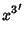(9)

The Determinant of the upper left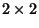Matrix in (3) is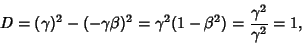(10)

so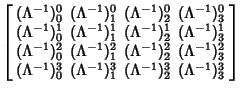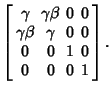A Lorentz transformation along the-axis can also be written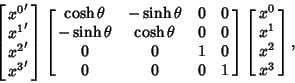(11)

where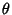is called the rapidity,(12)

and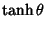(13)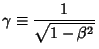(14)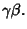(15)

References

Fraundorf, P. Accel-1D: Frame-Dependent Relativity at UM-StL.'' http://www.umsl.edu/~fraundor/a1toc.html.

Griffiths, D. J. Introduction to Electrodynamics. Englewood Cliffs, NJ: Prentice-Hall, pp. 412-414, 1981.

Morse, P. M. and Feshbach, H. The Lorentz Transformation, Four-Vectors, Spinors.'' §1.7 in Methods of Theoretical Physics, Part I. New York: McGraw-Hill, pp. 93-107, 1953.